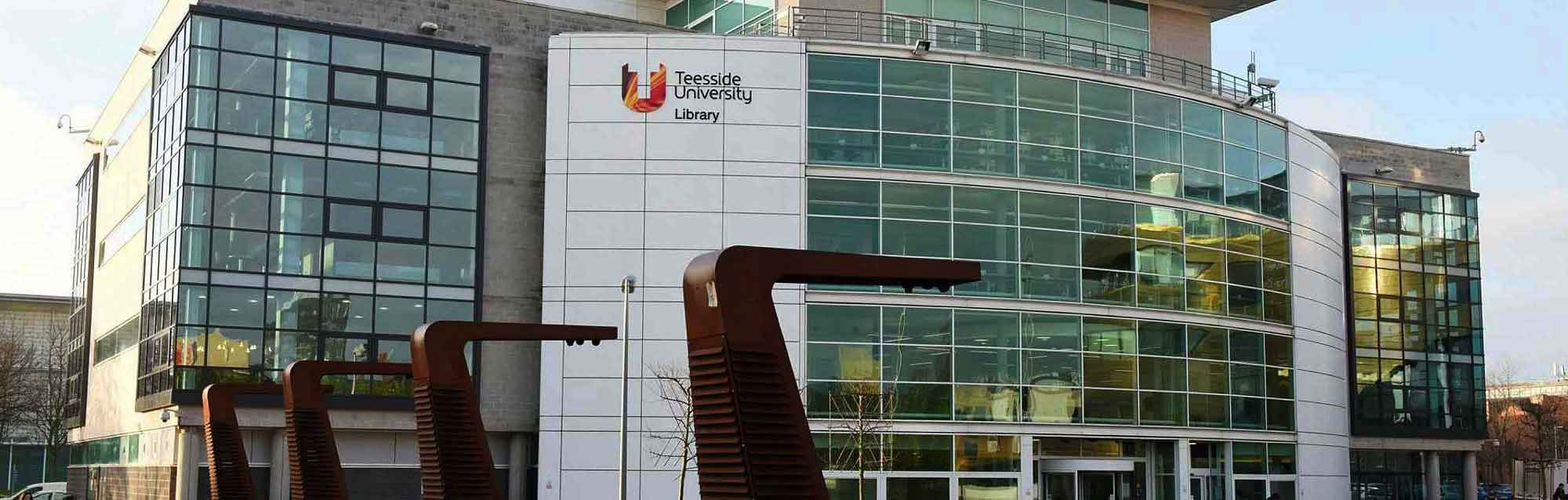Basic Mathematics

Course overview

This course improves your understanding of basicmathematical concepts to prepare you for a universitycourse. You study arithmetic, percentages, decimalsand equations, and go on to algebra and graphs. It’scrucial to master these skills if you intend to further youreducation at degree level.

Summer University 2019 has now finished. We will be running a range of short courses as part of Winter University in January 2020. Our Winter University 2020 brochure will be available in November - please register your details here if you would like to receive a brochure when it is available.

Where you study

Teesside University, Middlesbrough Campus

Course details

What you study

Module Aims
This module aims to develop the student’s skills in: basic arithmetic; methods of collecting, tabulating and displaying statistical data; the notation and techniques of basic algebra; the drawing and analysis of graphs of statistical and experimental data; and to boost the confidence of a student who needs to apply numeracy in future studies of their chosen profession.

Indicative Content
Number
- A review of basic arithmetic
- Calculations involving numerical fractions, decimals, percentages
- Units and conversion of units between different systems.
- Ratio and Proportion
- Application of arithmetic in everyday situations.

Statistics
- Methods of collection of statistical data
- Tabulation of statistical data
- Creation and interpretation of statistical charts and diagrams
- Calculation and analysis of basic statistics from a set of data

Algebra
- The concept of symbols representing numbers
- Introduction to the manipulation of algebraic expressions
- Solution of simple algebraic equations

Graphs
- Drawing and analysis of graphs from experimental data and algebraic functions

How you are assessed

End of course assessment: examination.

Entry requirements

Entry requirements

Prerequisite: Basic Understanding of numeracy.

Part-time

2019 entry

• Length: Credits: 10, Level: 3, Fees: £50.00